It’s one thing to read about something like this, and assume it’s valid, simply because it would be so easy to prove or disprove, and “someone would have by now” etc.

I first encountered this with Chuck Missler’s “Cosmic Codes” book, and have since found that it is well known, and is all over the WEB if you search.

I have been studying Gematria and Bible codes for many years now, and as a hobby coder have verified and expanded on much of it.

But it wasn’t until I wrote a Python script to perform the analysis below,

and actually did it myself, and saw the result on my screen in front of me right in my face,

that it really HIT ME !     This is REAL!

It could be a coincidence if just ONE Bible verse gave a special number – and that would be a valid counter-argument; I would be the first to say so!

But consider this:

The TWO verses in the Bible which deal with the BEGINNING of Creation, EACH give a “special” number.

And those two numbers are the most fundamental constants in all of physics.

The base of the natural logarithm, depicted as “e”, occurs all over in higher math and physics; it is intricately woven in to the “fabric” of the Universe, a true “creation” number!

Pi” is usually thought of as the ratio of the circumference of a circle to the diameter – but it shows up in math and physics all over the place – often dealing with phenomena that have nothing to do with circles or geometry!

These are two truly universal numbers, and didn’t the “math” (ie., the ‘blueprints, design, code, etc.) have to come before the actual “Creation” ?!

You could do these calculations by hand, but it would be very tedious and time consuming, also prone to error. Look at the numbers below – how large they are!

No, you need a computer.

And that’s just to discern them already being present. HOW could they have been put there by human intelligence and writing even today, let alone thousands of years ago?

In addition, do it in TWO different languages [Hebrew, Greek]; two ancient languages, the only two in human history that have numerical meanings to the letters.

To devise a method of letter-number conversions to equal specific special numbers, and then also be able to put those letters into a sentence or paragraph that actually means something and fits into a greater context?!

On top of that, do it thousands of years ago when there was no knowledge of mathematics beyond simple counting, no computers, etc.

There is no other explanation, it can’t be denied – these are the fingerprints of GOD !

So why?

The Prophecy in Daniel (12:4) and elsewhere implies a “hidden book” within the Scriptures that would be revealed in the Last Days, which we are clearly in now.

Could that be all these codes which have been discovered recently, and more every day, now with the computers we have had for only a few decades?

Could it be that as the End of Days approaches, we have grown arrogant in our knowledge, and proud in the works of our own hands, and have strayed from God.

And God, knowing this before hand, has put this all there as a way of reminding us – just at the time we need it?

Ponder it!

Also there is something unique about PI. It's not just a number, it's a concept.

It shows up when a lower dimension is encapsulated by a higher dimension. For example, PI = Circumference / Diameter, a one dimensional diameter line encapsulated by a two dimensional circle.

So what does all this mean?

In the original bible verse, it mentions 2 things, the Heavens and the Earth. A higher dimension and a lower dimension.

Another way of looking at it, the Heavens/Spiritual World and the Earth/Physical World(TimeSpace).

This would imply the the Physical world is an "encapsulated dimension" in the Spiritual world.

The closest concept we have to understanding what an encapsulated dimension is, would be something similar to Virtual Reality, or a Digital Simulation; a “projected game-space”.

Our Time/Space is a Virtual World encapsulated by the Spiritual world.   This is an amazing discovery. The secret of the universe is hidden in the first verse of the bible!

Ponder it!

So here it is – “PI” and the Natural Logarithm Base “e” are found in the Scriptures, written thousands of years ago!:

### Genesis 1:1   In the beginning God created the heaven and the earth.

In Hebrew: (a picture and 2 fonts, to increase the chances of your system displaying it correctly)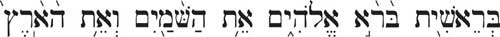בְּרֵאשִׁ֖ית בָּרָ֣א אֱלֹהִ֑ים אֵ֥ת הַשָּׁמַ֖יִם וְאֵ֥ת הָאָֽרֶץ

בראשית ברא אלהים את השמים ואת הארץ

If you examine the numerical values of each of the Hebrew letters, and the numerical value of the words, and apply them to this formula:

The number of letters x the product of the letters
The number of words x the product of the words

You get 3.1416 x 1017. The value of π to four decimal places! It is the Mantissa that is significant; the meaning of the exponent 1017 is yet to be discerned.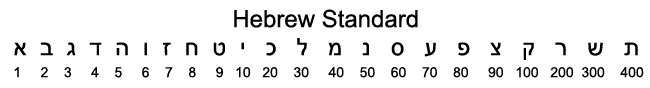### John 1:1   In the beginning was the Word, and the Word was with God, and the Word was God.

In Greek: (a picture and 2 fonts, to increase the chances of your system displaying it correctly)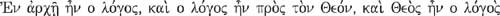ἐν ἀρχῇ ἦν ὁ λόγος, καὶ ὁ λόγος ἦν πρὸς τὸν θεόν, καὶ θεὸς ἦν ὁ λόγος

εν αρχηι ην ο λογος και ο λογος ην προς τον θεον και θεος ην ο λογος

This time if you take the numerical value of each of the Greek letters, and the numerical value of the words, and apply them to the same formula:

The number of letters x the product of the letters
The numberof words x the product of the words

You now get 2.7183 x 1040, the value of e. Again, It is the Mantissa that is significant; the meaning of the exponent 1040 is yet to be discerned, although "40" does have considerable meanings in Scripture.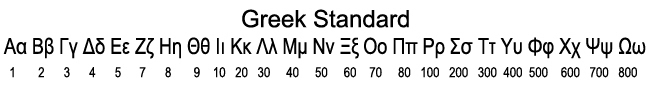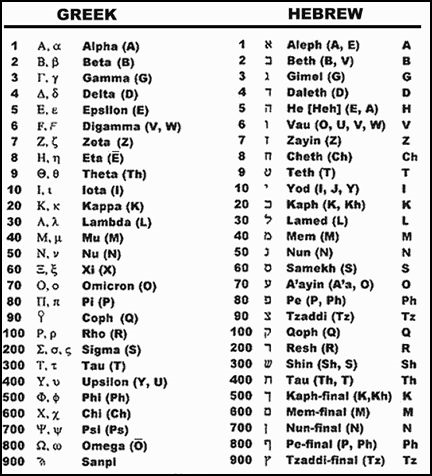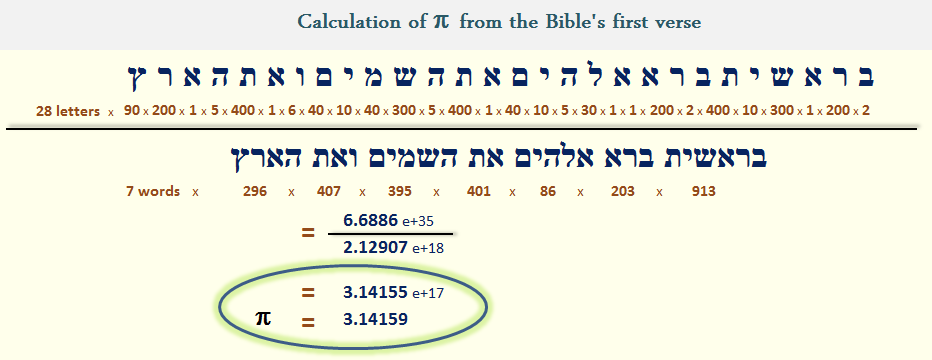For the Genesis 1:1 in Hebrew:

[Hebrew is very tricky coding – it reads right to left and everything is backwards!]

My program when run displays this:

Enter a word or phrase: בראשית ברא אלהים את השמים ואת הארץ

ב = 2

ר = 200

א = 1

ש = 300

י = 10

ת = 400

ב = 2

ר = 200

א = 1

א = 1

ל = 30

ה = 5

י = 10

ם = 40

א = 1

ת = 400

ה = 5

ש = 300

מ = 40

י = 10

ם = 40

ו = 6

א = 1

ת = 400

ה = 5

א = 1

ר = 200

ץ = 90

Number of Letters = 28

Product of Letters = 23887872000000000000000000000000000

The number of letters x the product of the letters = 668860416000000000000000000000000000

בראשית = 913

ברא = 203

אלהים = 86

את = 401

השמים = 395

ואת = 407

הארץ = 296

Number of Words = 7

Product of Words = 304153525784175760

The number of Words x the product of the words = 2129074680489230320

Result = 668860416000000000000000000000000000 / 2129074680489230320 = 3.1415545078310995e+17

Actual Pi = 3.141592653589793

[correlation = 0.9999878578278917]

Verse Gematria = 2701

For the John 1:1 in Greek:

My program when run displays this:

Enter a word or phrase: εν αρχηι ην ο λογος και ο λογος ην προς τον θεον και θεος ην ο λογος

ε = 5

ν = 50

α = 1

ρ = 100

χ = 600

η = 8

ι = 10

η = 8

ν = 50

ο = 70

λ = 30

ο = 70

γ = 3

ο = 70

ς = 200

κ = 20

α = 1

ι = 10

ο = 70

λ = 30

ο = 70

γ = 3

ο = 70

ς = 200

η = 8

ν = 50

π = 80

ρ = 100

ο = 70

ς = 200

τ = 300

ο = 70

ν = 50

θ = 9

ε = 5

ο = 70

ν = 50

κ = 20

α = 1

ι = 10

θ = 9

ε = 5

ο = 70

ς = 200

η = 8

ν = 50

ο = 70

λ = 30

ο = 70

γ = 3

ο = 70

ς = 200

Number of Letters = 52

Product of Letters = 8436251456259110830080000000000000000000000000000000000000000000000000000000

The number of letters x the product of the letters = 438685075725473763164160000000000000000000000000000000000000000000000000000000

εν = 55

αρχηι = 719

ην = 58

ο = 70

λογος = 373

και = 31

ο = 70

λογος = 373

ην = 58

προς = 450

τον = 420

θεον = 134

και = 31

θεος = 284

ην = 58

ο = 70

λογος = 373

Number of Words = 17

Product of Words = 949302241399839184297857869760000000

The number of Words x the product of the words = 16138138103797266133063583785920000000

Result = 438685075725473763164160000000000000000000000000000000000000000000000000000000 / 16138138103797266133063583785920000000 = 2.718312812196422e+40

Actual e = 2.718281828459045

[correlation = 1.0000113982799916]

Verse Gematria = 3627

Note the huge numbers! Do this by hand? Yeah right. Conceive this and write it down – 2000 and 3000+ years ago? I don’t think so!

Now what more proof do you need !?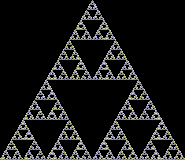10/01/2018:   I have found other instances of PI and E in the Bible, as well as the Fine Structure Constant!   Check it out!

.